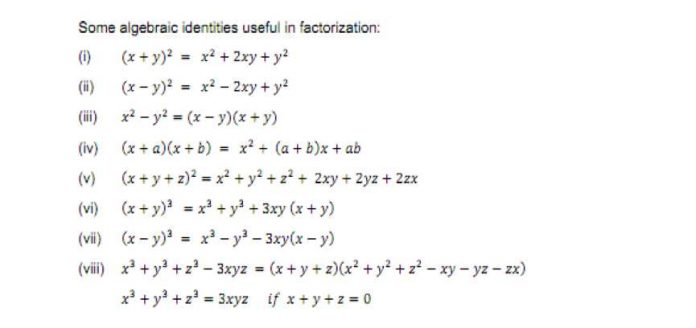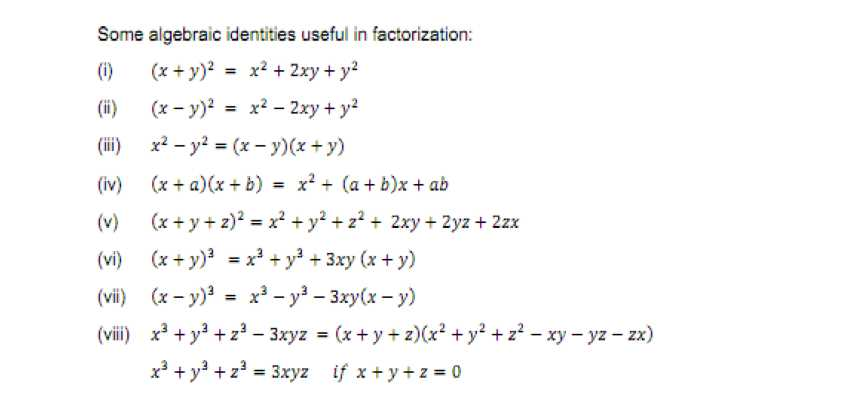# Chapter 2 Polynomials Notes for Class 9th Math

0
2188CHAPTER – 2 POLYNOMIAL

1. Polynomials in one Variable
2. Zeroes of a Polynomial
3. Remainder Theorem
4. Factorisation of Polynomials
5. Algebraic Identities
• Constants: A symbol having a fixed numerical value is called a constant.
• Variables: A symbol which may be assigned different numerical values is known as variable.
• Algebraic expressions: A combination of constants and variables. Connected by some or all of the operations +, -, X and is known as algebraic expression.
• Terms: The several parts of an algebraic expression separated by ‘+’ or ‘-‘ operations are called the terms of the expression.
• Polynomials: An algebraic expression in which the variables involved have only nonnegative integral powers is called a polynomial.
1. 5x2 – 4x2 – 6x – 3 is a polynomial in variable x.

3

1. (ii) 5 + 8x2 + 4x-2 is an expression but not a polynomial.

Polynomials are denoted by p(x), q(x) and r(x)etc.

• Coefficients: In the polynomial x3 + 3x2 + 3x +1, coefficient of x3, x2, x are1,3,3 respectively

and we also say that +1 is the constant term in it.

• Degree of a polynomial in one variable: In case of a polynomial in one variable the highest

power of the variable is called the degree of the polynomial.

• Classification of polynomials on the basis of degree.

Degree Polynomial Example

1. 1 Linear x +1, 2x + 3etc.
2. 2 Quadratic ax2 + bx + c etc.
3. 3 Cubic x3 + 3x2 +1 etc. etc.

Classification of polynomials on the basis of no. of terms

Polynomial & Examples. Monomial – S3Sx31Yetc.

3

No. of terms

(i) 1

Binomial – (3 + 6x), (x – 5y) etc.

(ii) 2

(iii) 3

Trinomial- 2x2 + 4x + 2 etc. etc.

• Constant polynomial: A polynomial containing one term only, consisting a constant term is called a constant polynomial the degree of non-zero constant polynomial is zero.
• Zero polynomial: A polynomial consisting of one term, namely zero only is called a zero polynomial. The degree of zero polynomial is not defined.
• Zeroes of a polynomial: Let p(x) be a polynomial. If p(a) =0, then we say that is a zero of the polynomial of p(x).
• Remark: Finding the zeroes of polynomial p(x) means solving the equation p(x)=0.
• Remainder theorem: Let f (x) be a polynomial of degree n > I and let a be any real

number. When f(x) is divided by (x – a) then the remainder is f (a)

• Factor theorem: Let f(x) be a polynomial of degree n > 1 and let a be any real number.
1. If f (a) = 0 then (x – a) is factor of f (x)
2. If (x – a) is factor of f (x)then f (a) = 0
• Factor: A polynomial p(x) is called factor of q(x) divides q(x) exactly.
• Factorization: To express a given polynomial as the product of polynomials each of degree less than that of the given polynomial such that no such a factor has a factor of lower degree, is called factorization.Previous articleChapter 1 Number Systems Notes for Class 9th Maths